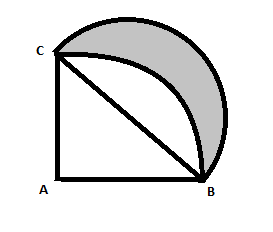Courses
Courses for Kids
Free study material
Free LIVE classes
MoreLIVE
Join Vedantu’s FREE Mastercalss

# In the following figure $ABC$ is a quadrant of a circle of radius 14cm and a semicircle is drawn with $BC$ as diameter. Find the area of the shaded region.Verified
360k+ views
Hint: At first find out the length of $BC$ by using Pythagoras theorem. Then find out the area of the triangle and area of the quadrant. Find out the area of the semicircle. Lastly subtract the area of the non shaded part of the semicircle from the area of the semicircle to get the area of the shaded part.

Let us take the figure first:We have to find out the area of the shaded region. Now if we look at the figure very carefully,
The area of the shaded region = area of the semicircle – area of the non shaded part of the semicircle.
To find out the area of the semicircle we need to find out the length of $BC$ , the diameter of the semicircle.
We can clearly see that $BC$ is the hypotenuse of the right angle triangle $ABC$ .
So, we can use Pythagoras theorem, that is:
$B{{C}^{2}}=A{{B}^{2}}+A{{C}^{2}}$ , as $AB,BC$ are the radius of the circle $AB=AC=14cm$ .
\begin{align} & \Rightarrow B{{C}^{2}}={{14}^{2}}+{{14}^{2}} \\ & \Rightarrow B{{C}^{2}}=196+196 \\ & \Rightarrow B{{C}^{2}}=392 \\ & \Rightarrow BC=\sqrt{392}cm \\ \end{align}
$BC$ is the diameter of the semicircle. So the radius of the semicircle is $\dfrac{BC}{2}$ .
That is $\dfrac{\sqrt{392}}{2}cm$ .
Area of the semicircle is:
$=\dfrac{1}{2}\times \pi {{r}^{2}}$ , r is the radius of the semicircle. Let us put $\pi =\dfrac{22}{7}$ .
\begin{align} & =\dfrac{1}{2}\times \dfrac{22}{7}\times {{\left( \dfrac{\sqrt{392}}{2} \right)}^{2}} \\ & =\dfrac{11}{7}\times \dfrac{392}{4}=\dfrac{11\times 56}{4}=11\times 14=154c{{m}^{2}} \\ \end{align}

Now$ABC$ is a quadrant of a circle of radius 14.
We know that the area of quadrant of a circle is:
$=\dfrac{1}{4}\times \pi {{r}^{2}}$ , r is the radius of the circle.
$=\dfrac{1}{4}\times \pi \times {{14}^{2}}$
Put, $\pi =\dfrac{22}{7}$
\begin{align} & =\dfrac{1}{4}\times \dfrac{22}{7}\times 14\times 14=154c{{m}^{2}} \\ & \\ \end{align}
We know that area of a right angle triangle is = $\dfrac{1}{2}\times base\times height$
Here base is $AC$ , height is $AB$ .
So the area of the triangle is:
$=\dfrac{1}{2}\times 14\times 14=7\times 14=98c{{m}^{2}}$
Area of the non shaded part of the semicircle = area of the quadrant of the circle – area of the right angle triangle.
\begin{align} & =\left( 154-98 \right)c{{m}^{2}} \\ & =56c{{m}^{2}} \\ \end{align}
Now we have the area of the semicircle and the area of the non shaded part of the semicircle.
So the area of the shaded region is = area of the semicircle – area of the non shaded part of the semicircle.
$=154-56=98c{{m}^{2}}$
Therefore the area of the shaded portion is $98c{{m}^{2}}$ .

Note: Here we have to remember the formula of area of a right angle triangle.
If we know the formula of the area of a circle we can easily calculate the area of the quadrant of a circle.
Area of the quadrant of a circle is $\dfrac{1}{4}\times \pi {{r}^{2}}$ , r is the radius of the circle.
Area of a semicircle or we can say half of the circle is $\dfrac{1}{2}\times \pi {{r}^{2}}$ .
Last updated date: 17th Sep 2023
Total views: 360k
Views today: 3.60k